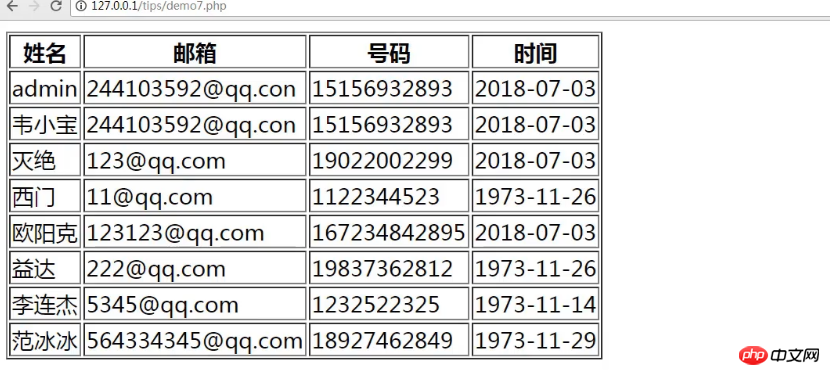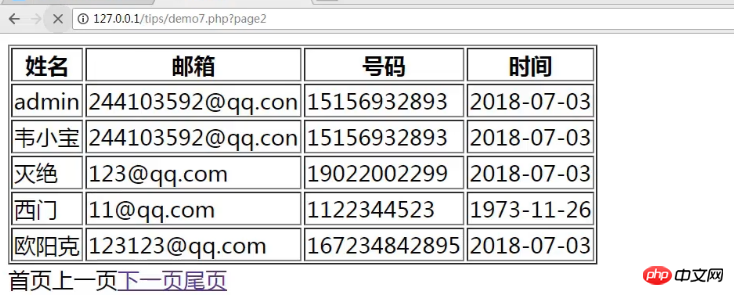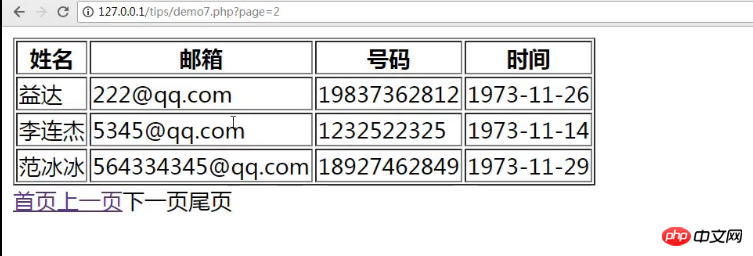# PHP怎么实现分页功能？（图文+视频教程）

PHP实现分页功能的操作，对于PHP新手来说或许有一定的难度。下面我们会通过详细的代码示例，为大家一一解说。希望对需要的朋友有一定的帮助。

PHP实现分页功能的完整代码示例如下：

``````<?php

\$host = '127.0.0.1';

\$user = 'root';

\$dbName = 'qiye';

\$pdo = new PDO("mysql:host=\$host;dbname=\$dbName", \$user, \$password);

\$sql = "select * from user";

\$data = \$pdo->query(\$sql)->fetchAll();

\$num = 5;

\$count = count(\$data);

\$w = ceil(\$count / \$num);

\$page = isset(\$_GET['page']) ? \$_GET['page'] : 1;

//var_dump(\$page);

\$offset = (\$page - 1) * \$num;

//var_dump(\$offset);

\$sql = "select * from user limit \$offset,{\$num}";

\$data = \$pdo->query(\$sql)->fetchAll();

\$p = (\$page == 1) ? 0 : (\$page - 1);

\$n = (\$page == \$w) ? 0 : (\$page + 1);

?>

<!doctype html>

<html lang="en">

<meta charset="UTF-8">

<title>Document</title>

<body>

<form action="">

<table border="1">

<tr>

<th>姓名</th>

<th>邮箱</th>

<th>号码</th>

<th>时间</th>

</tr>

<?php foreach (\$data as \$v): ?>

<tr>

<td><?php echo \$v['email']; ?></td>

<td><?php echo \$v['phone']; ?></td>

<td><?php echo date("Y-m-d", \$v['time']); ?></td>

</tr>

<?php endforeach; ?>

</table>

<?php

if (\$page==1){

echo "首页";

}else{

echo "<a href='?page=1'>首页</a>";

}

if (\$p){

echo "<a href='?page={\$p}'>上一页</a>";

}else{

echo "上一页";

}

if (\$n){

echo "<a href='?page={\$n}'>下一页</a>";

}else{

echo "下一页";

}

if(\$page== \$w){

echo "尾页";

}else{

echo "<a href='?page={\$w}'>尾页</a>";

}

?>

</form>

</body>

</html>``````PHP跳转分页原理：如果\$p即当前页数不是在首页也就是不等于1时，我们就将当前页数减去1，即可实现点击上一页的跳转操作；如果\$n即当前页数不是在尾页也就是不等于总页数时，我们就将当前页数加上1，即可实现点击下一页的跳转操作；如果要实现点击首页或者尾页时，实现直接跳转操作，只要将\$p或\$n的值设置为1或者总页数即可。×